### Home > CC4 > Chapter 10 > Lesson 10.2.3 > Problem10-108

10-108.
1. Solve each inequality below. Represent the solutions on number lines. 10-108 HW eTool (CPM). Homework Help ✎

1. 7x – 2 < 3 + 2x

2.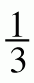x ≥ 2

3. 3(2m – 1) – 5m ≤ –1

4. 2k + 3 ≤ 2k + 1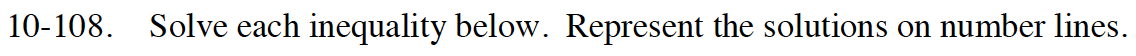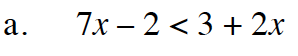Find the boundary point.
7x − 2 = 3 + 2x

Solve for x.
5x = 5
x = 1

Test numbers on both sides of 1.

7(0) − 2 < 3 + 2(0) 7(2) − 2 < 3 + 2(2)
0 − 2 < 3 + 0 14 − 2 < 3 + 4
−2 < 3 12 < 7
True False

x < 1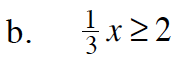Follow the steps in part (a).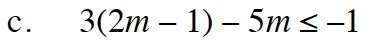Follow the steps in part (a).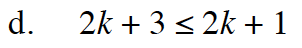Follow the steps in part (a).

Does this inequality have a solution?

Use the eTool below to represent the solutions on number lines.
Click the link at right for the full version of the eTool: Int1 10-108 HW eTool